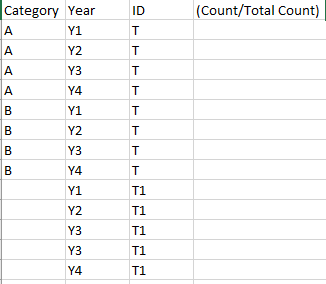# New to Qlik Sense

If you’re new to Qlik Sense, start with this Discussion Board and get up-to-speed quickly.

Announcements
QlikWorld 2023, a live, in-person thrill ride. Save \$300 before February 6: REGISTER NOW!
cancel
Showing results for
Did you mean:Contributor

## Calculating Percentage of Total by dimension and Year

Hi,

I want to calculate percentage of Total (ID) according to category and Year. I am using this formula

NUM(
ALT(
Count({< Year=, Year = {"<=\$(=Max(Year))"},ID = {'T'}>}ID)
/
Count({Year=, Year = {"<=\$(=Max(Year))"},< ID = {'T'}>} Total ID)
,0)
,'#,##0%').

I want to see trend in my Bar chart for Each year and By using this formula, it giving me percentage by all Year. I want percentage of total for each category and year.

 Category Year Id A 2019 T B 2020 T B 2021 T B 2022 T

Labels (1)
• ### Chart

2 RepliesEmployee

You cannot use set analysis for this. Set analysis is not evaluated row-by-row in the chart. It is like a selection for all rows in the measure.

I assume that Category and Year are your dimensions, so that you have one row per combination of Category and Year. Further, I assume that you want to calculate the number for the row, divided by the total for the entire year. I.e. respect the year, but total over all Categories.

Then you could use

Count(ID) / Count(total <Year> ID)Contributor
Author

Hi Henric,

Thanks for the reply.  This Solution also gave the same result. I have added samplet

It should Calculate Total Percentage (A/ (A+B) ) for Y1, (B/A+B) for Y1, (A/(A+B) for Y2, (B/A+B) for Y2 and so on  where Id= T.Tags
Community Browser